Learn All Concepts of Chapter 3 Class 12 Matrices - FREE. Check - Matrices Class 12 - Full video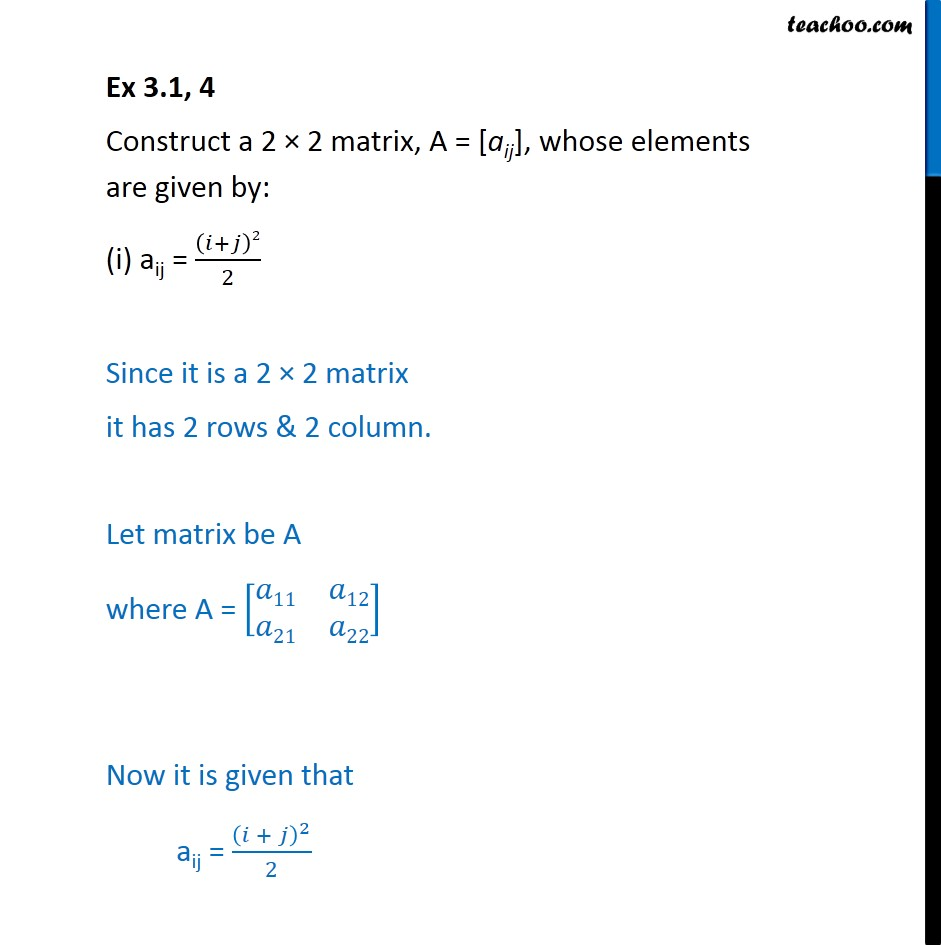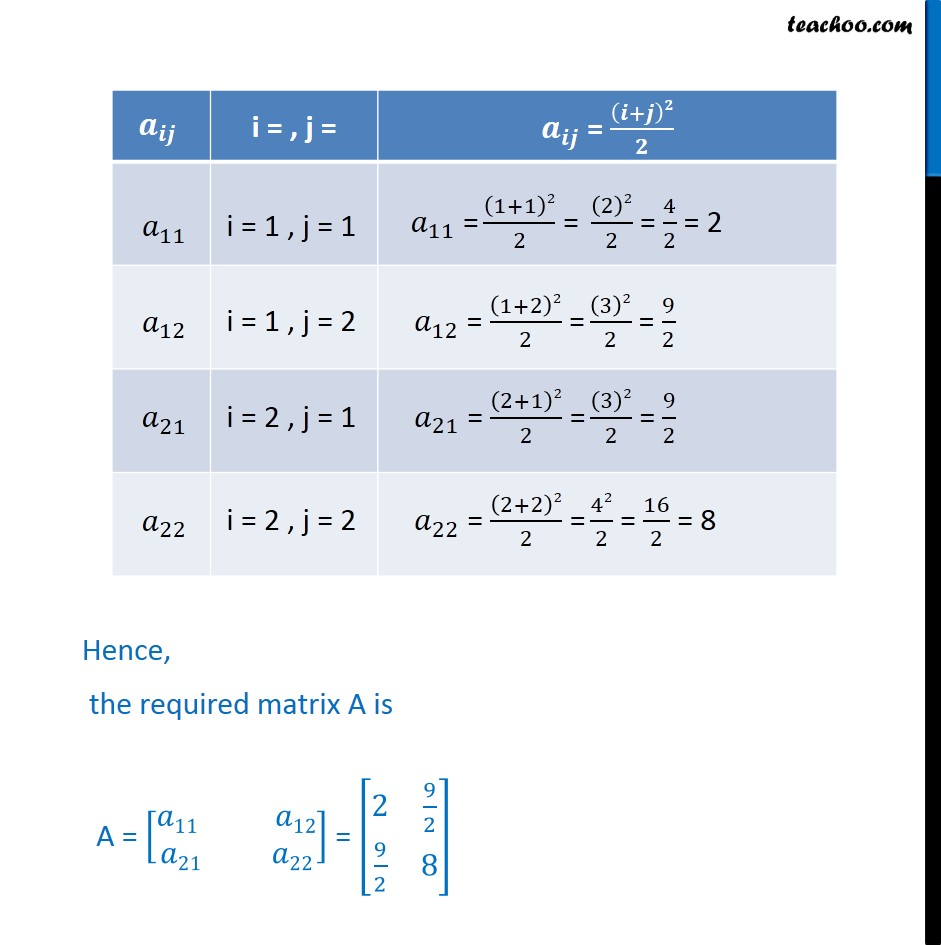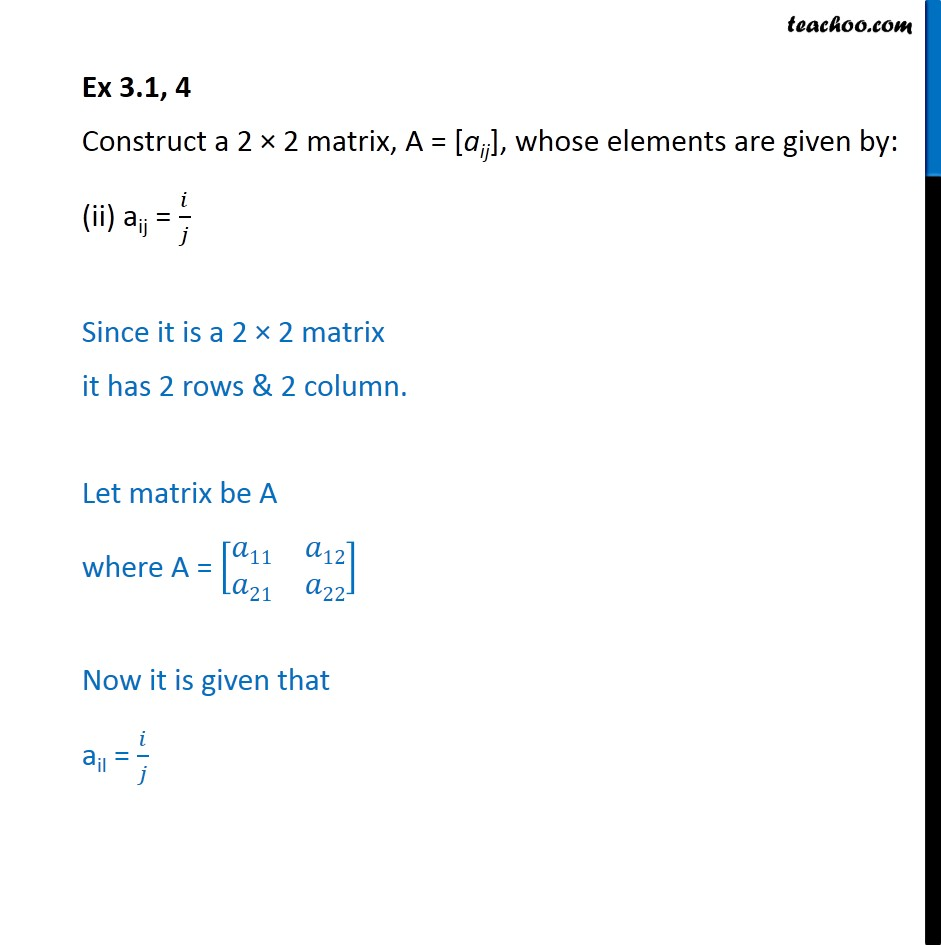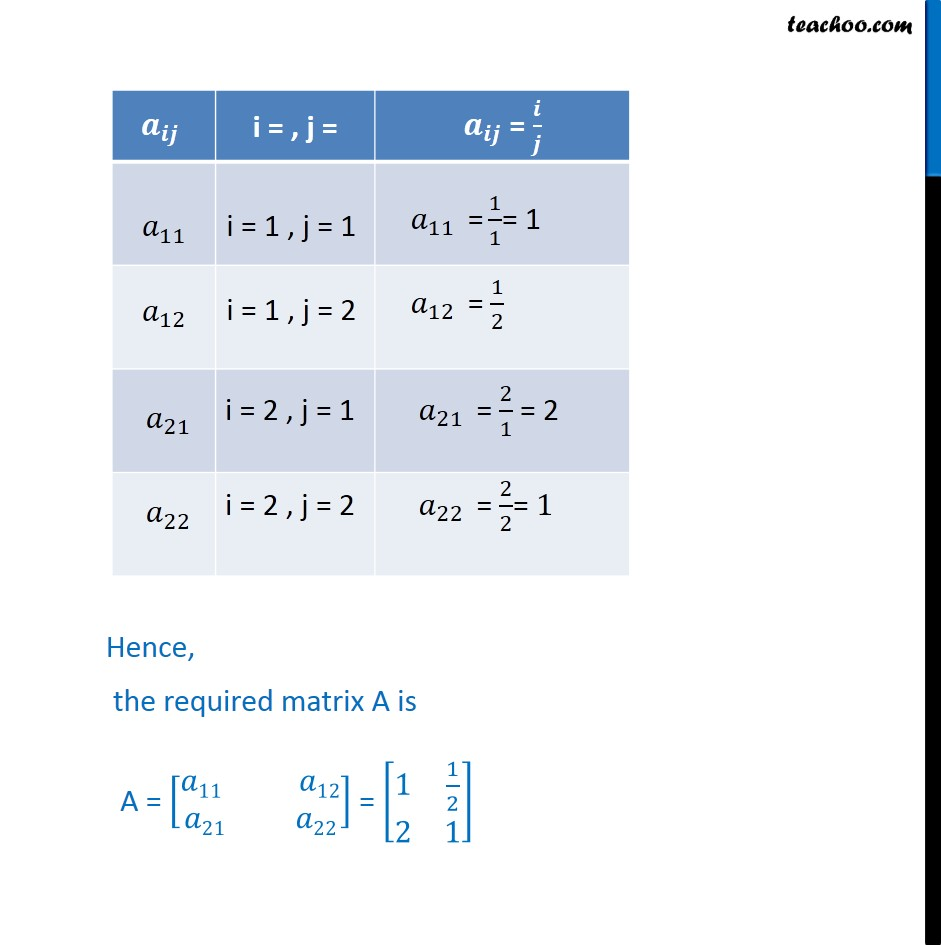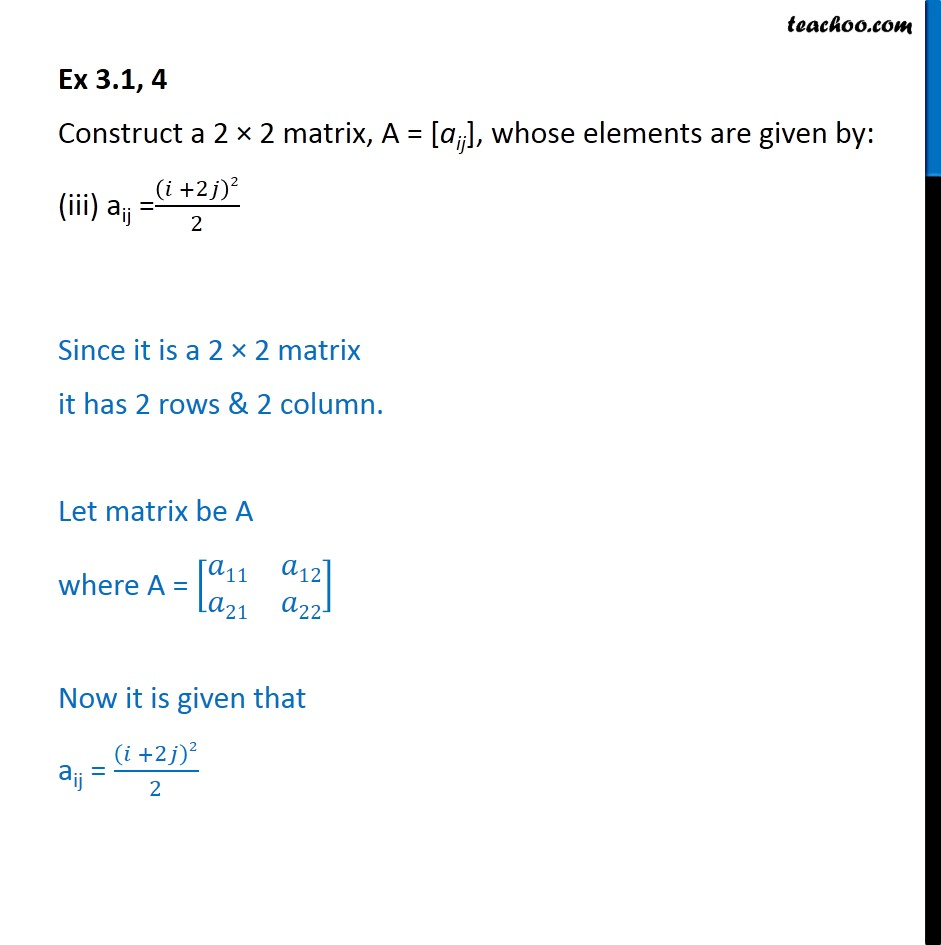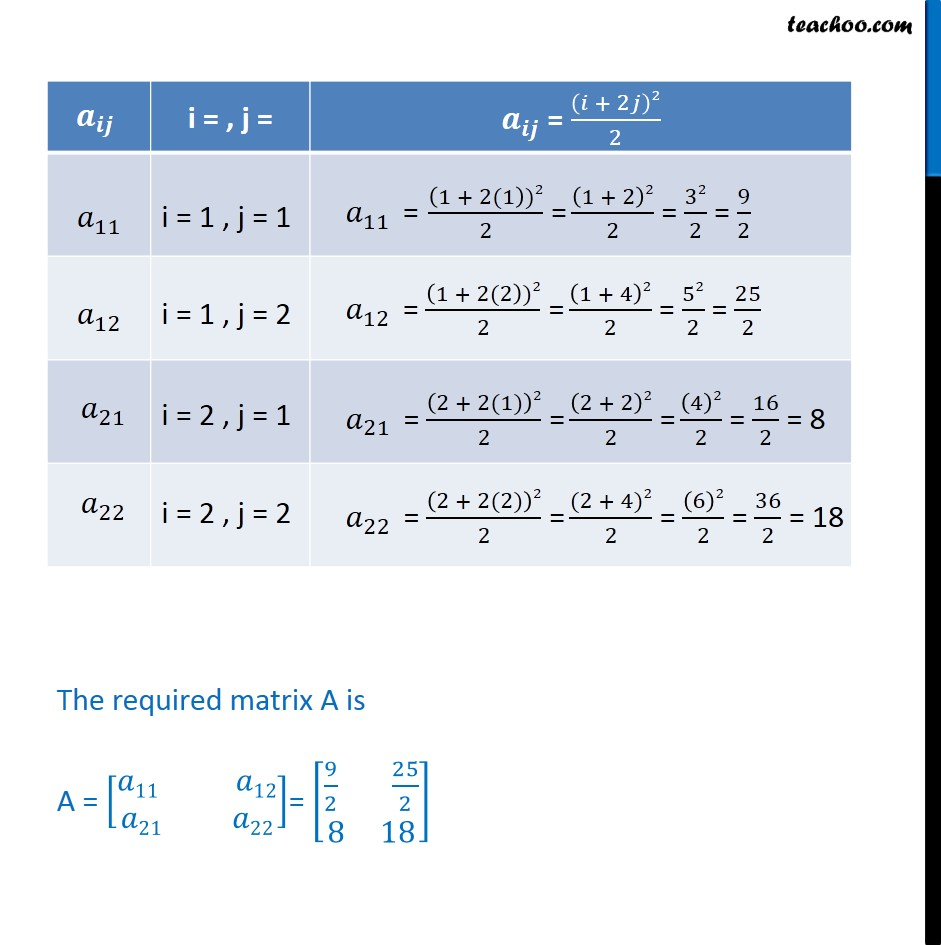1. Chapter 3 Class 12 Matrices
2. Serial order wise
3. Ex 3.1

Transcript

Ex 3.1, 4 Construct a 2 × 2 matrix, A = [aij], whose elements are given by: (i) aij = (𝑖+𝑗)2/2 Since it is a 2 × 2 matrix it has 2 rows & 2 column. Let matrix be A where A = [■8(𝑎11&𝑎12@𝑎21&𝑎22)] Now it is given that aij = (𝑖 + 𝑗)^2/2 Ex 3.1, 4 Construct a 2 × 2 matrix, A = [aij], whose elements are given by: (ii) aij = 𝑖/𝑗 Since it is a 2 × 2 matrix it has 2 rows & 2 column. Let matrix be A where A = [■8(𝑎11&𝑎12@𝑎21&𝑎22)] Now it is given that ail = 𝑖/𝑗 Ex 3.1, 4 Construct a 2 × 2 matrix, A = [aij], whose elements are given by: (iii) aij =(𝑖 +2𝑗)2/2 Since it is a 2 × 2 matrix it has 2 rows & 2 column. Let matrix be A where A = [■8(𝑎11&𝑎12@𝑎21&𝑎22)] Now it is given that aij = (𝑖 +2𝑗)2/2 The required matrix A is A = [█(𝑎11 𝑎12@𝑎21 𝑎22)]= [█(9/2 25/2@8 18)]

Ex 3.1

Chapter 3 Class 12 Matrices
Serial order wise